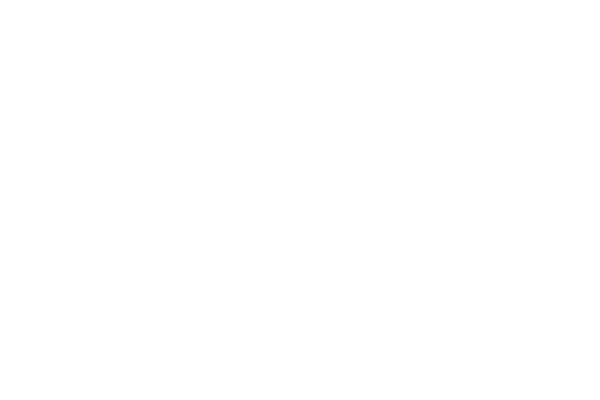# Nonlinear Crystals: LTB

DescriptionLTB (Lithium Triborate or LBO2) is a nonlinear crystal that is commonly used in laser technology for frequency doubling, tripling, and mixing. It has a relatively high nonlinear coefficient, wide transparency range, and good thermal and mechanical properties, making it a popular choice for many applications.

In frequency doubling, LTB is used to convert a laser beam from one frequency to another by doubling its frequency. This process can be used to generate laser light in the ultraviolet region, which is useful for many applications such as spectroscopy, microscopy, and material processing.

In frequency tripling, LTB is used to triple the frequency of a laser beam, resulting in laser light in the visible range. This process is used for applications such as laser-induced fluorescence and optical communications.

LTB can also be used in frequency mixing, where two different laser beams are combined in the crystal to produce a new frequency that is the sum or difference of the original frequencies. This process is useful for generating laser light in new frequency ranges that may not be accessible with other methods.

 Transparency range, µm 0.16 - 3.6 Point group 4mm Space group I41cd Lattice parameters, Å a = 9.479; c = 10.297 Density, g/cm3 2.44 Mohs hardness 4.5 Refractive indexes: at 266 nm no = 1.6664; ne = 1.6031 at 532 nm no= 1.6139, ne = 0.5564 at 1064 nm no = 1.5980, ne = 1.5432 Non-linear coefficient, pm/V d31 = 0.12 Walk off 1.9° at 532 nm Temperature acceptance, °C x cm 3.5 Angle acceptance 1.52° x cm1/2 Temperature SHG tuning (Δ λ/Δ T) 9.3 x 10E-3 nm/°C Dielectric constant ε11T/ε0 = 82.61; ε11S/ε0 = 78.80; ε33T/ε0 = 87.92; ε33S/ε0 = 71.45; Thermal expansion coefficient a11 = 11.1 x 10-6 x °K-1; a33 = 3.74 x 10-6 x °K-1 Elastic stiffness, 1010 N/m2 C11E = 13.5; C33E = 5.68; C12E = 0.375 C44E = 5.85; C13E = 3.35; C55E = 14.67; Piezoelectric stress constants, C/m2 e15 = 0.45; e31 = 0.209; e33 = 0.928

Order Form

Drag and drop files here or Browse
*You can upload your specification file here. Max 5MB. Allowed File Types: JPG, PNG, PDF